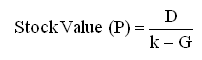CFA CFA Level 1 can not work out the formula….

# can not work out the formula….

• Author
Posts
• 0

Hi there,
I am new to this forum. I would be grateful if anyone could help to answer this question
“if required yield on undated 8% Treasury with par value £100 is 6%, what is its price?” this question is bugging since yesturday evening. What is the formula to use in this situation

Aramis

• 5

Correct, you need to know what the investor’s required rate of return is, however (now someone correct me if I’m wrong) I believe the question is asking you two pieces:

(a) calculate what the investor’s rate of return is, then
(b) calculate the intrinsic value via Gordon Growth.

My initial thoughts are to try to work out part (a) using CAPM, but then again I feel it’s missing something…..

• 5

Zee,

thank you for your help. I am still confused as to how you found “required return k” . I am aware of the formula what I find difficult is the way you rearrange formula to find x. Is this trial & error method??? I am feeling like a special need person to ask this question but 1020=25/(x-0.18)
how to rearrange to get x?

• 4

I believe you are correct. Essentially you can ignore the par value and look at the required rate of return versus what payment is being received,

i.e., for an asset that pays \$8, what would an investor be willing to pay if their required rate of return is 6% p.a…. therefore \$8/6% = \$8/0.06 = \$133.33

(not sure where the pound key is on my keyboard, so I’ve used dollars instead)

• 4

Hey @aramis77Where
D=dividend=25 pence
k=required return=x
G=growth rate=18%
P=price=1020 pence

feeding it all in you’ll get @dr_pain28’s working:

1020=25/(x-0.18)
x=20.45098%

• 4

Zee,

nice one. Thank you

• 3

@Aramis77 – two disclaimers, been a while since I did LI and I don’t work in Fixed Income. By undated, do you mean it’s essentially a perpetuity? Would it not be C/R in that case? i.e. c.£133 in your example?

• 3

Jwa,
spot on mate. How did you come to this figure? could you share the formula please?

• 3

Dr_Pain28
Result is correct. Thank you for you input. My question is now
how did you find x in your formula? I have tried different method can not understand how you arrived at 20.4509

• 2

Well as I say I am by no means certain but I think in this case you simply divide the coupon (£8, assuming annual payment) by the required yield (0.06)… probably too simple to be correct.

• 2

Thank you mitch895 and Jwa.
could you help out on this one maybe, being very cheeky sorry
“using gordon growth, what is expected return on following share:
price 1020p
dividend per share 25p
sales 160m
beta 0.9
market capital 400m
operating profit 15m
expected growth rate 18%

I tried Gordon growth formula, did not work, too many elements missing…

• 2

@Aramis777.I see were you are going with your thought process @mitch895, maybe the question is asking us to reverse engineer the required rate of return that would price the stock at \$1020 (Since we don’t have enough information to compute the CAPM.); although I am not sure if this is what the question is asking. Doing this I came to a rate of return of 20.45098%.

1020=25/(x-0.18)
x=20.45098%

Again I’m not sure if this is what the question is asking you to do, the information from the problem seemed short and incomplete. I hope this helps.

• 1

1020=25/(x-0.18)

x-0.18=25/1020

x=25/1020+0.18

x=20.45098%